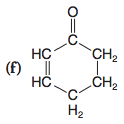Problem: Rewrite each of the following using bond-line formulas:

FREE Expert Solution
80% (217 ratings)
Problem Details

Rewrite each of the following using bond-line formulas:What scientific concept do you need to know in order to solve this problem?

Our tutors have indicated that to solve this problem you will need to apply the Skeletal Structure concept. You can view video lessons to learn Skeletal Structure Or if you need more Skeletal Structure practice, you can also practice Skeletal Structure practice problems .

What is the difficulty of this problem?

Our tutors rated the difficulty of Rewrite each of the following using bond-line formulas: ... as low difficulty.

How long does this problem take to solve?

Our expert Organic tutor, Jonathan took half a minute to solve this problem. You can follow their steps in the video explanation above.

What textbook is this problem found in?

Our data indicates that this problem or a close variation was asked in . You can also practice practice problems .